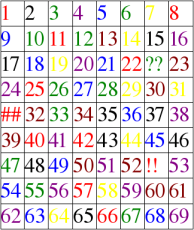## Designing a Magic Trick

Imagine a magician who comes on stage and performs the following magic trick:

He asks someone in the audience to think of a two-digit number, then subtract the sum of its digits. He waves his wand and guesses that the result is divisible by nine. Ta-Da!

This is not magic. This is a theorem. To make it magical we need to disguise the theorem.First, there are many ways to hide the fact that we subtract the sum of the digits. For example, we can ask to subtract the digits one by one, while chatting in between. It is better to start with subtracting the first digit. Indeed, if we start with subtracting the second digit, the audience might notice that the result is divisible by 10 and start suspecting that some math is involved here. You can be more elaborate in how you achieve the subtraction of the sum of digits. For example, subtract twice the first digit, then the second, then add back the original number divided by 10.

Second, we need to disguise that the result is divisible by 9. A nice way to do this is implemented in the online version of this trick. The website matches the resulting number to a gift that is described on the page in pale letters. Paleness of letters is important as it is difficult to see that the same gift reappears in a pattern. In my work with students I use the picture on the left. At the end I tell them, “Ta-Da! the resulting number is blue.” Here is the full sized version of the same picture that you can download.

My students are too smart. They see through me and guess what is going on. Then I ask them the real question, “Why do I have some cells with question marks and other symbols?” To give you a hint, I can tell you that the symbols are there for the same reason some blue numbers are not divisible by 9.

Share:

### One Comment

1. #### ano:

Because otherwise all the blue numbers will be along a straight line (diagonal), or fall into some easily recognizable pattern?